# 5 watt power amplifier measurements

Under construction...

Wideband linear PA by DL2EWN (FunkAmateur 07/2008 and 08/2008).The amplifier has been characterized for different driver stage supply voltage (Vd), final stage supply voltage (Vf) and final stage standby current (If):

## Vd=12V, Vf=12V If=700 mA

The fundamental and harmonics output levels versus input power, with a 10 MHz sinewave input, is shown in the graph below: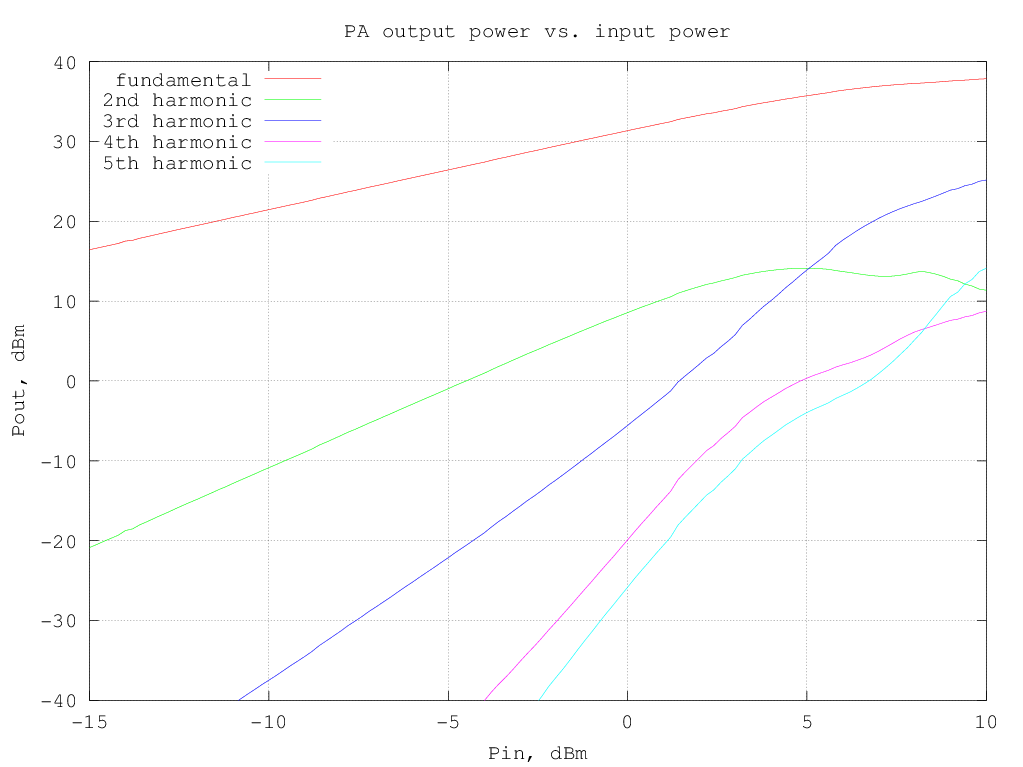RF output and DC current drawn from the supply versus input power (10 MHz sinewave) are shown in the following graph: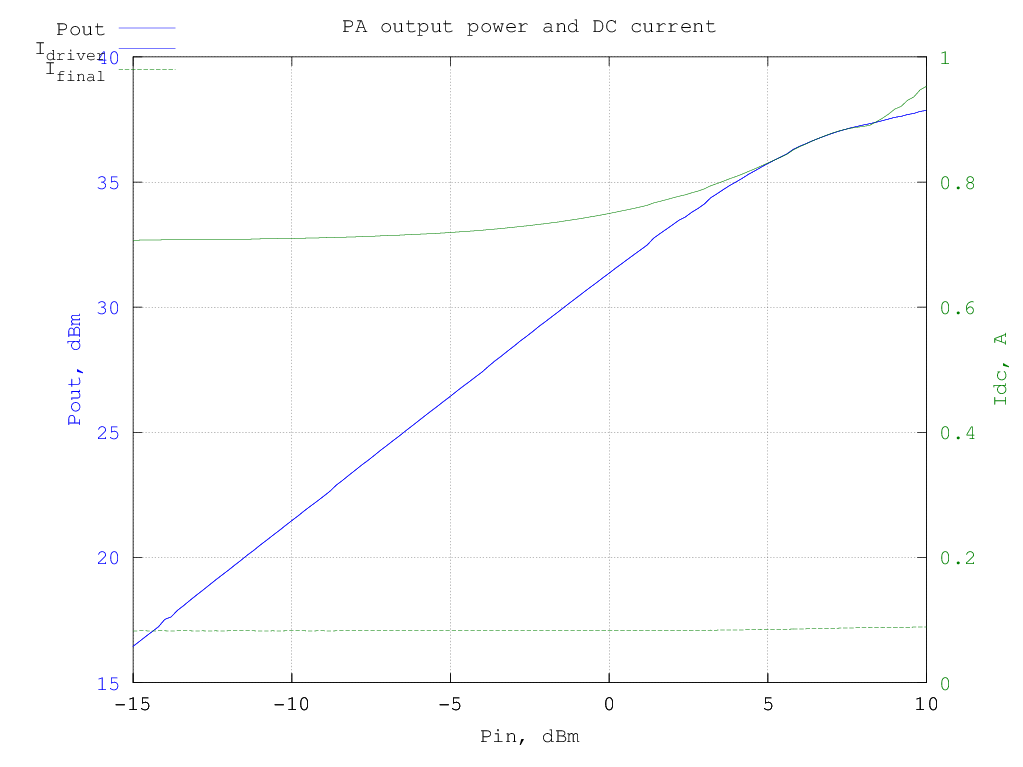and the resulting efficiency is shown in the graphs below (data are the same for the two graphs, in the first the input and output power are in dBm and in the second they are in watt):

The third-order intermodulation products amplitude w.r.t the output power per-tone is shown in the graph below (to obtain the actual [average] output power multiply the value by two, to have the PEP, multiply by 4):IMD products vs. tone spacing at 3.5 W PEP output## Vd=12V, Vf=13.8V, If=700 mA

The fundamental and harmonics output levels versus input power, with a 10 MHz sinewave input, is shown in the graph below:RF output and DC current drawn from the supply versus input power (10 MHz sinewave) are shown in the following graph: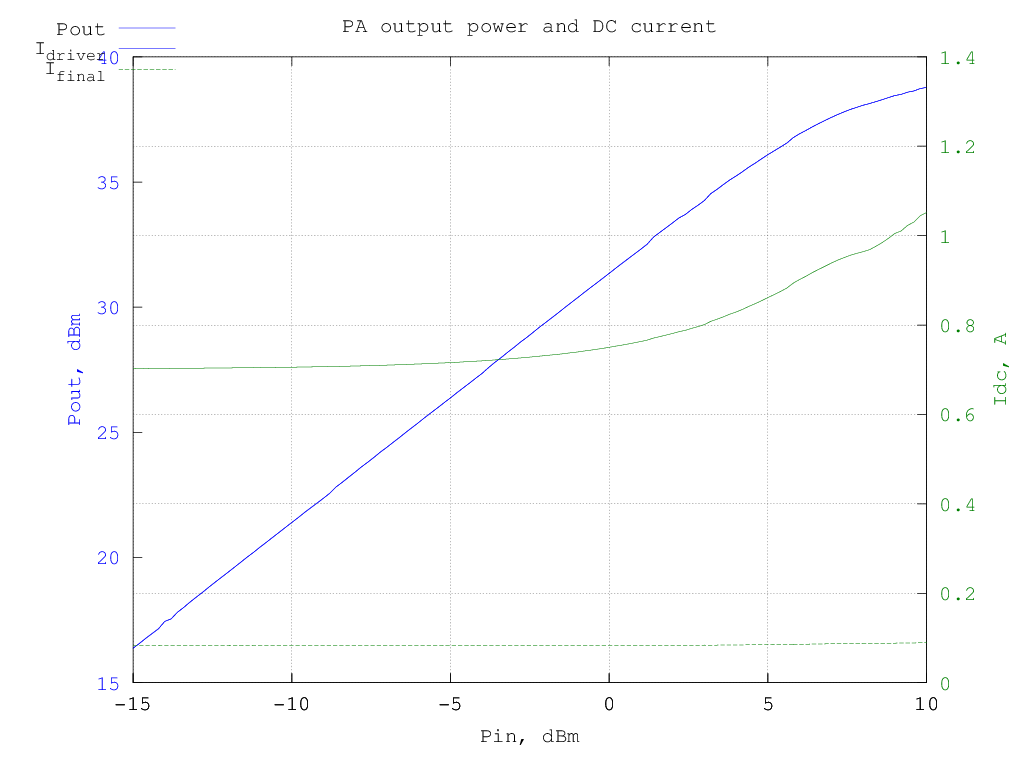and the resulting efficiency is shown in the graphs below (data are the same for the two graphs, in the first the input and output power are in dBm and in the second they are in watt):

The third-order intermodulation products amplitude w.r.t the output power per-tone is shown in the graph below (to obtain the actual [average] output power multiply the value by two, to have the PEP, multiply by 4):## Vd=12V, Vf=15V, If=700 mA

The fundamental and harmonics output levels versus input power, with a 10 MHz sinewave input, is shown in the graph below: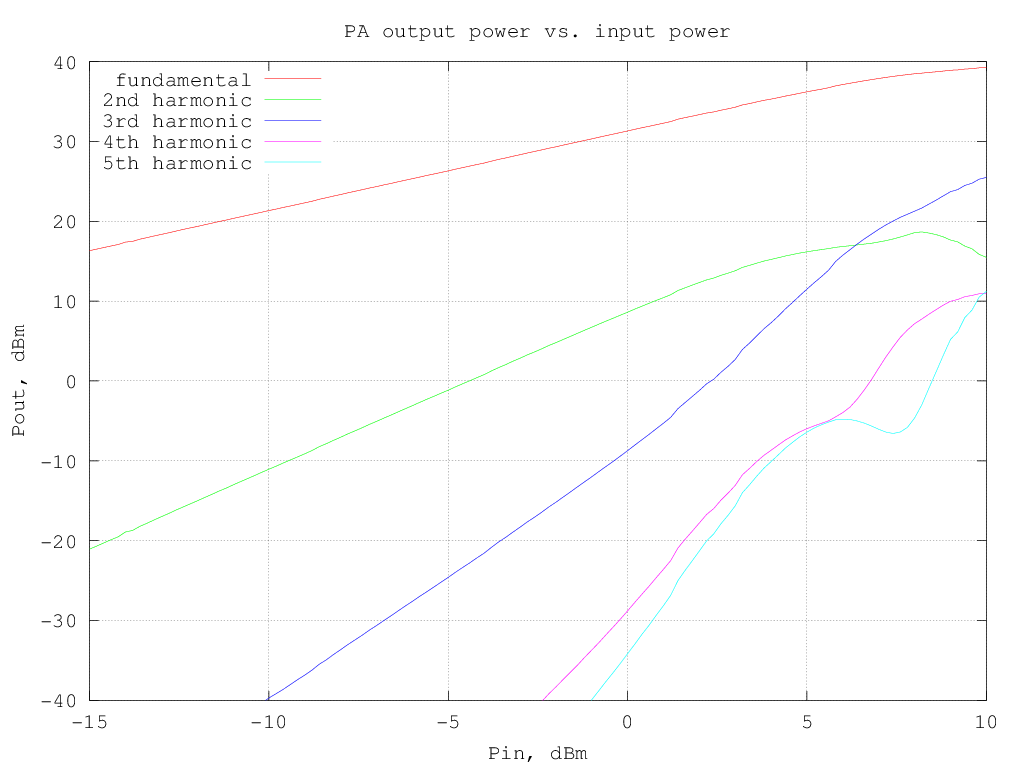RF output and DC current drawn from the supply versus input power (10 MHz sinewave) are shown in the following graph: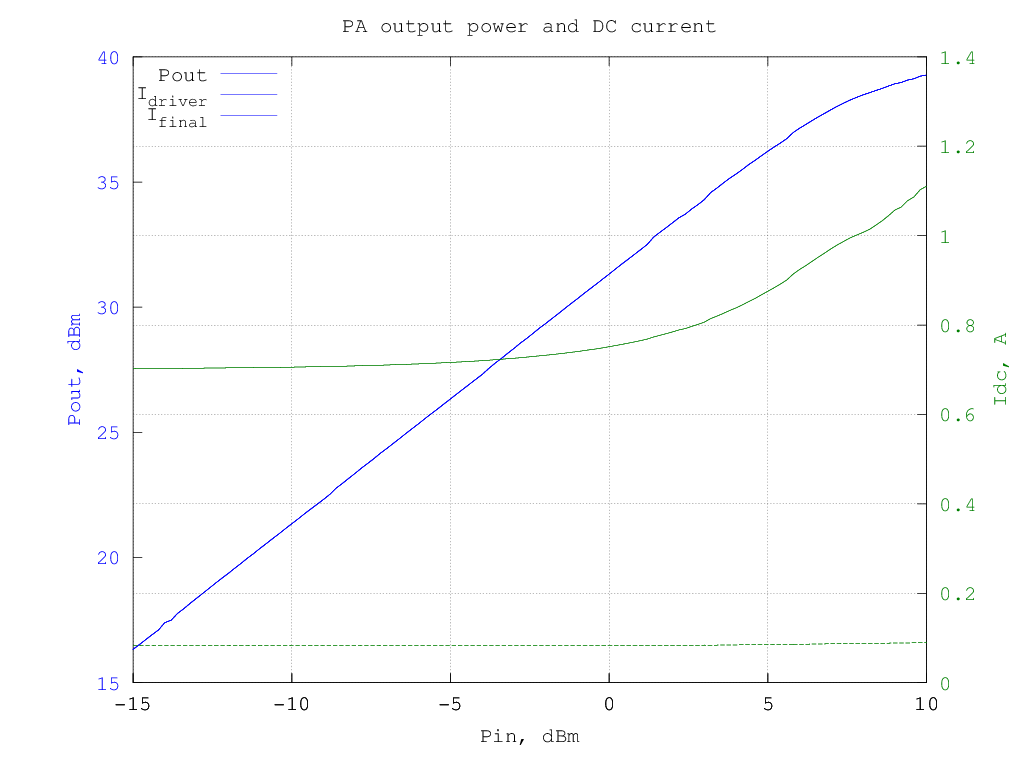and the resulting efficiency is shown in the graphs below (data are the same for the two graphs, in the first the input and output power are in dBm and in the second they are in watt):

The third-order intermodulation products amplitude w.r.t the output power per-tone is shown in the graph below (to obtain the actual [average] output power multiply the value by two, to have the PEP, multiply by 4):